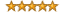# Interview Questions for Business Analysts and Systems Analysts

Recent Interview Questions | Search | Subscribe (RSS)

?
INTERVIEW QUESTION:

# What is Cost Benefit Analysis (CBA)?// 37217 Views // 4 Additional Answers & Comments

Cost Benefit Analysis is a technique used to determine if the financial benefits of a project outweigh the associated cost of undertaking the project in the first place.  For a short term project where the benefit may be an immediate one-time cash windfall this may be as simple as subtracting the total of all the project cost from the total of all of the project benefits.  If the total is positive, then the project may be worth completing.

Project Duration = 2 months
Project Costs = \$50,000
Immediate Project Benefits = \$75,000
\$75,000 - \$50,000 = \$25,000

However, project costs and benefits rarely result in such a simple example.  Project costs and benefits often occur over time.   For this reason, Cost Benefit Analysis should consider all project cost and benefits in terms of the present value (PV) of money.

To determine the present value of a future cost or benefit we discount the value of the dollars to account for time.  To calculate the Present Value we use the formula PV = FV /[(1 + i)^t].

PV = Present Value
FV = Future Value
i = Discount Rate
t = time

In our example, if the \$50,000 cost was incurred immediately and the \$75,000 benefit was realized 3 years in the future, using a 5% discount rate would result in the following:

PV = \$75,000 / [(1 + .05)^3] = \$64,787.82
\$64,787.82 - \$50,000 = \$14,787.82

The net benefit of this project is now clearly less than originally thought.

Taking this a step further, consider the example where we have multiple cashflows (costs and benefits) over time.

@ T0, Cost = -\$25,000
@ T1, Cost = -\$25,000, Benefit = \$15,000
@T2, Benefit = \$30,000
@T3, Benefit = \$30,000

By subtracting the present value of future costs from the present value of future benefits, we can arrive at the Net Present Value (NPV) of the costs and benefits for each year of the project.   The sum of all NPV calculations will give us the NPV of the entire project.

NPV = FVben – FVcost / [(1 + i)^t]
NPV0 = \$0 - \$25,000 / [(1 + .05)^0] = -\$25,000
NPV1 = \$15,000 - \$25,000 / [(1 + .05)^1] = -\$9,523.81
NPV2 = \$30,000 - \$0 / [(1 + .05)^2] = \$27,210.88
NPV3 = \$30,000 - \$0 / [(1 + .05)^3] = \$25,915.13

For a total of \$18,602.20 in benefit.

## RATE THIS TOPIC

Olya posted on Monday, February 9, 2009 3:58 PM
could you give any reference to literature where this technique is addressed?Sanat posted on Tuesday, May 19, 2009 11:36 AM
Look sensible ...
But how do u decide the discount rate.
Can you describe little about it?

Thanks.zarfman posted on Thursday, February 11, 2010 5:47 PM

The timing of cash outflows, cash inflows, discount rates, reinvestment rates are hard to predict. Project overruns can destroy the projects cost benefits. I suspect many cost benefit analysis done before our current economic crisis are now in shambles.

Sometimes the benefit is simply conforming to some law or regulation - you don't get sued.

Sometimes the project is postulated on a reduction in staff - did that really happen?

If one continuously recalculates the cost benefit over time - what does one do if the cost overwhelms the benefit?

I suspect that it boils down to how well one can predict the future - not so easy to do.

Regards,

zarfman - the old accounting and finance dude.JBUTLER posted on Friday, March 26, 2010 3:52 PM
The discount would be the projected rate of growth that has to exist for one dollar deposited now to be one dollar in x years in the future (the present value of a dollar available x years in the future)Only registered users may post comments.

## Select ModernAnalyst Content

brought to you byenabling practitioners & organizations to achieve their goals using:

## Community Resources

Copyright 2006-2023 by Modern Analyst Media LLC